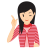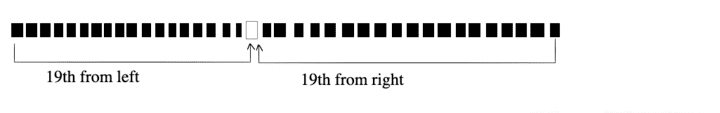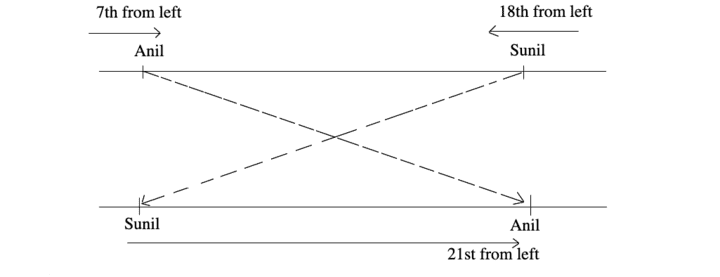# Number, Ranking and Time Sequence Test

Number, ranking and time sequence test is based on arrangement of characters/ persons/ objects in a particular order based on some specific parameters.

In this chapter, we deal with questions which have either a sequence of numbers, position of a person/object in a row/queue or time sequence test accompanied by some conditions.

## Type of Questions

Generally three types of questions based on number, ranking and time sequence test are asked in various competitive exams.

Type #1:
Number Test

In this type of questions, a number/a set of numbers/a series of digits is given and the candidates are asked to find out digits following certain given conditions.

Example: 1   How many 5's are there in the following sequence which are preceded by 3 but not followed by 8?

4 5 8 3 2 7 3 5 1 7 8 9 3 5 8 3 1 3 5 2

(a) 4           (b) 3          (c) 2          (d) 1

Solutions (c)  4 5 8 3 2 7 3 5 1 7 8 9 3 5 8 3 1 3 5 2

So, it is clear from above that three are two 5's in the given sequence which are preceded by 3 but not followed by 8.

Example: 2   How many even numbers are there in the following series of numbers, each of which is preceded by an odd number, but not followed by an even number?

5 3 4 8 9 7 1 6 5 3 2 9 8 7 3 5

(a) 0           (b) 1           (c) 2           (d) 3

Solutions (d)  5  3  4  8  9  7  1  6  5  3  2  9  8  7  3  5

There are three such even numbers 6, 2 and 8 each of which is preceded by an odd number and not followed by an even number.

Directions (Example Nos. 3-4) Following questions are based on the five three digit numbers given below.

519      364      287      158      835

Example: 3   If the positions of the first and the third digits within each number are interchanged, which of the following will be the third digit of the second lowest number?

(a) 9          (b) 5           (c) 7           (d) 8

Solutions (d) According to the question, after interchanging the position of the first and third digits, new numbers are 519 => 915, 364 => 463, 287 => 782, 158 => 851, 835 => 538, so second lowest number = 538.

Hence, third digit of the second lowest number is 8.

Example: 4   Which of the following is the difference between the second digit of the lowest and the highest of these numbers?

(a) 3                (b) 1

(c) 2                (d) 0

Solutions (c) According to the question,
Highest number = 835
Lowest number = 158Difference between the second digit of the lowest and the highest number = 5 - 3 = 2

Example: 5   The position of how many digits in the number  351462987  will remain unchanged after the digits are rearranged in ascending order within the number?

(a) None

(b) One

(c) Two

(d) Three

Solutions (c) Given number       3 5 1 4 6 2 9 8 7

After rearrangement                  1 2 3 4 5 6 7 8 9

Hence, there are two numbers, 4 and 8 which remain unchanged after the rearrangement.

Example: 6   The positions of the first and the sixth digit in the number  5109238674  are interchanged. Similarly, the positions of the second and seventh digit are interchanged and so on. Which of the following will be the third digit from the right end after the rearrangement?

(a) 9                (b) 0

(c) 6                (d) 3

Solutions (c) Given number = 5109238674

After interchanging the digits,
new number = 3867451092

Hence, third digit from the right end = 0

Type #2:
Ranking Test

In this type of questions, generally, the rank of a person either from the top/bottom or from right/left or the total number of persons is to be find out based on conditions given in question.Important Formulae Related to Ranking Test

The position or rank can be calculated with the help of following formulae

1. Total number of persons in a row = (Rank of a person from upper or left end) + (Rank of that person from lower or right end) -1

[because that particular place counts two times]

2. Rank of a person from upper or left end = (Total number of persons in row) - (Rank of that person from lower or right end) + 1

3. Rank of a person from lower or right end = (Total number of persons in row) - (Rank of that person from upper or left end) + 1

4. In case of interchanging of positions

(a) Total number of persons =
[Initial position of 1st person + Interchanged position of 2nd person] - 1

(b) New position of 2nd person =
[Difference in the two positions of 1st person] + [Initial position of 2nd person]

Example: 7   In a class, boys stand in a single row. One of the boy is nineteenth in order from both the ends. How many boys are there in the row?

(a) 27         (b) 37         (c) 38         (d) 39

Solutions (b) According to the question,Clearly, number of boys in the row = (18 + 18 +1) = 37

Alternate Method (Using Formula 1)

Total number of boys in the row = 19 + 19 - 1 = 37

Example: 8   In a class of 42 students, Mahesh's rank is 16th from the bottom. What is his rank from the top?

(a) 25th      (b) 26th      (c) 24th      (d) 27th

Solutions (d) Number of students ahead of Mahesh = 42 - 16 = 26

Now, Mahesh's rank from top = 26 + 1 = 27

So, Mahesh's rank is 27th from the top.

Alternate Method (Using Formula 2)Mahesh's rank from the top = 42 - 16 + 1 = 27

Example: 9   Anu and Vinay are ranked seventh and eleventh, respectively from the top in a class of 31 students. What will be their respective ranks from the bottom in the class?

(a) 20th and 24th           (b) 24th and 20th

(c) 25th and 21st            (d) None of these

Solutions (c) Number of students after Anu in the class = (31 - 7) = 24

Now, Anu's rank from bottom = 24 + 1 = 25

So, Anu is 25th from the bottom.

Number of students after Vinay in the class = (31 - 11) = 20

Now, Vinay's rank from bottom = 20 + 1 = 21

So, Vinay is 21st from bottom = 20 + 1 = 21

Alternate Method (Using Formula 3)Anu's rank from the bottom = 31 - 7 + 1 = 25

Vinay's rank from the bottom = 31 - 11 + 1 = 21

Example: 10   In a row of students, Anil is 7th from left, while Sunil is 18th from right. Both of them interchanged their positions such that Anil becomes 21st from left. What will be the total number of students in the class?

(a) 38      (b) 33      (c) 31      (d) 30

Solutions (a)Total number of students = [Initial position of Sunil + Interchanged position of Anil] -1

= [18 + 21] = 38

Example: 11   Mohan is taller than Shyam but shorter than Ramesh. Ramesh is taller than Rajat but shorter than Gautam. If Shyam is taller than Rajat, then who is the shortest among all?

(a) Gautam      (b) Rajat      (c) Shyam      (d) Ramesh

Solutions (b) From given information,

Ramesh > Mohan > Shyam         .....(i)

Gautam > Ramesh > Rajat         ......(ii)

Shyam > Rajat                          ......(iii)

Combining Eqs. (i), (ii) and (iii), we get,

Gautam > Ramesh > Mohan > Shyam > Rajat

Hence, Rajat is the shortest among all.

Type #3:
Time Sequence Test

In this type of questions, candidates are required to find out a particular day or time based on some given conditions.

To solve such type of problems, the candidate must have a basic knowledge regarding calendar and clock.

Example: 12   Sunita leaves her house at 20 min to seven in the morning, reaches Vineeta's house in 25 min, they finish their breakfast in another 15 min and leave for their office which takes another 35 min. At what time, did they leave Vineeta's house to reach their office?

(a) 7 : 40 am            (b) 7 : 20 am

(c) 7 : 45 am            (d) 8 : 15 am

Solutions (b) Sunita leaves her house at 6 : 40 am.

She reaches Vineeta's house in 25 min,
i.e. 6 : 40 + 0 : 25 at 7 : 05 am.

Both leave for office, 15 min after 7 : 05 am
i.e. 7 : 05 + 0 : 15 at 7 : 20 am.

Example: 13   Kamala would like to complete all her work before 10 : 00 pm in order to watch an important TV programme. She has 40 min assignment in each of her five prepared subjects. What is the least time at which she can start and still complete her home work in the time for the programme?

(a) 6 : 40 pm           (b) 6 : 30 pm

(c) 7 : 10 pm           (d) 7 : 20 pm

Solutions (a) Time required to complete the homework
= 5 x 40 = 200 min = 3 h 20min

Now, the required time = 10 : 00 - 03 : 20

= 06 : 40pm

Example: 14   If every second Saturday and all Sundays are holidays in a 30 days month beginning on Saturday, then how many working days are there in that month?

(a) 20         (b) 21         (c) 22         (d) 23

Solutions (d) As, the month begins with Saturday.

So, 2nd, 9th, 16th, 23rd, 30th are Sundays, while 8th and 22nd are second Saturday. Thus, there are 7 holidays in all.Number of working days = 30 - 7 = 23

Example: 15   Samant remembers that his brother's birthday is after 15th but before 18th of February, while, his sister remembers that her brother's birthday is after 16th but before 19th of February. On which date, of February, is Samant's brother's birthday?

(a) 16th            (b) 18th

(c) 19th            (d) 17th

Solutions (d) According to Samant, 16, 17

According to Possible date 16, 17 17, 18

Here, 17th is common.

Hence, brother's birthday is on 17th.

LearnFrenzy provides you lots of fully solved "Number, Ranking and Time Sequence Test" Questions and Answers with explanation.